It's a Big Fat PandaHover over the thumbnail for a full-size version.

Author nDEAVOUR author:ndeavour cool inspired n-art panda pandamonium rated 2009-07-15 2009-07-15 4 by 41 people. \$It's a Big Fat Panda#nDEAVOUR#inspired#00000000000000000000000000000000000000000000000000000000000000000000000000000000000000000000000000000000000000000000000000000000000000000000000000000000000000000000000000000000000000000000000000000000000000000000000000000000000000000000000000000000000000000000000000000000000000000000000000000000000000000000000000000000000000000000000000000000000000000000000000000000000000000000000000000000000000000000000000000000000000000000000000000000000000000000000000000000000000000000000000000000000000000000000000000000000000000000000000000000000000000000000000000000000000000000000000000000000000000000000000000000000000000000000000000000000000000000000000000000000000000000000000000000000000000000000000000000000000000|5^36,564!10^264,294!10^262,294!10^262,295!10^263,295!10^266,295!10^264,295!10^264,292!10^264,292!10^262,292!10^262,293!10^262,294!10^262,294!10^264,294!10^264,294!10^264,291!10^264,292!10^265,294!10^265,296!10^263,296!10^186,312!10^186,312!10^186,312!10^186,312!10^186,310!10^186,310!10^184,312!10^187,313!10^188,313!10^188,309!10^185,309!10^185,311!10^185,312!10^185,312!3^267,293!10^253,298!10^260,302!10^266,311!10^273,316!10^282,317!10^286,310!10^280,305!10^272,300!10^269,296!10^250,286!10^250,294!10^266,287!10^277,299!10^280,321!10^270,317!10^255,303!10^186,299!10^184,308!10^181,312!10^176,317!10^174,324!10^179,333!10^187,328!10^187,322!10^185,317!10^188,302!10^185,332!10^183,327!10^265,139!10^268,139!10^272,141!10^274,141!10^277,141!10^278,142!10^280,144!10^284,144!10^287,145!10^290,146!10^294,149!10^296,151!10^299,154!10^302,160!10^304,161!10^307,163!10^310,166!10^311,168!10^312,173!10^312,175!10^312,179!10^312,180!10^312,185!10^306,189!10^303,191!10^298,193!10^292,195!10^287,195!10^282,193!10^280,191!10^276,189!10^274,189!10^272,187!10^270,185!10^267,185!10^264,183!10^262,181!10^259,179!10^257,177!10^255,173!10^251,171!10^251,167!10^251,165!10^251,161!10^251,156!10^251,153!10^252,151!10^254,148!10^258,142!10^258,142!10^262,140!10^266,140!10^269,143!10^266,145!10^261,148!10^261,151!10^257,154!10^257,156!10^257,160!10^257,165!10^258,170!10^261,172!10^264,170!10^266,165!10^266,162!10^268,158!10^270,155!10^274,150!10^279,150!10^280,152!10^277,156!10^271,161!10^271,162!10^271,164!10^268,172!10^268,173!10^268,183!10^271,181!10^273,176!10^281,169!10^281,166!10^285,160!10^290,154!10^288,155!10^278,161!10^283,165!10^294,159!10^296,162!10^297,166!10^298,167!10^300,169!10^302,172!10^302,176!10^302,180!10^300,181!10^294,184!10^292,186!10^287,187!10^285,187!10^283,184!10^283,180!10^283,177!10^288,175!10^292,175!10^293,172!10^292,165!10^292,161!10^282,168!10^272,175!10^131,156!10^134,153!10^137,153!10^140,155!10^142,159!10^143,161!10^147,167!10^148,171!10^151,172!10^155,174!10^159,174!10^160,174!10^160,179!10^160,185!10^160,188!10^156,195!10^156,200!10^153,203!10^153,211!10^153,212!10^153,213!10^146,207!10^146,203!10^143,199!10^133,159!10^132,159!10^132,159!10^130,162!10^130,166!10^130,168!10^130,171!10^132,175!10^132,177!10^134,181!10^136,183!10^138,186!10^138,187!10^138,191!10^140,193!10^142,196!10^145,199!10^146,193!10^148,189!10^152,182!10^148,180!10^143,180!10^143,172!10^140,166!10^140,167!10^136,172!10^143,178!10^147,180!10^246,367!10^246,369!10^244,371!10^242,371!10^240,372!10^239,372!10^238,372!10^237,372!10^235,372!10^230,372!10^228,372!10^226,374!10^222,375!10^218,375!10^214,376!10^214,376!10^214,376!10^214,376!10^217,377!10^219,377!10^221,378!10^223,379!10^225,379!10^227,380!10^229,380!10^231,380!10^233,378!10^234,377!10^235,375!3^290,377!3^303,366!3^168,171!3^169,171!3^174,169!3^176,167!3^181,165!3^183,161!3^185,161!3^187,159!3^190,155!3^191,155!3^196,153!3^197,151!3^200,147!3^202,147!3^206,143!3^210,139!3^215,137!3^219,135!3^226,129!3^168,175!3^166,175!3^164,179!3^164,182!3^164,183!3^164,189!3^164,197!3^164,202!3^159,211!3^157,215!3^155,221!3^153,226!3^151,226!3^149,230!3^147,235!3^147,241!3^144,243!3^145,248!3^145,248!3^145,251!3^145,254!3^145,258!3^146,263!3^147,268!3^145,272!3^145,277!3^145,282!3^145,288!3^145,292!3^145,293!3^143,298!3^143,299!3^143,308!3^143,309!3^146,315!3^146,315!3^150,322!3^151,327!3^152,332!3^154,338!3^156,342!3^158,346!3^160,349!3^163,353!3^167,359!3^172,366!3^173,367!3^178,370!3^182,371!3^187,373!3^190,373!3^144,310!3^149,320!3^151,321!3^270,389!3^265,390!3^265,391!3^263,392!3^259,392!3^259,394!3^259,395!3^255,396!3^253,397!3^250,397!3^248,397!3^245,398!3^243,398!3^241,398!3^238,399!3^233,400!3^223,401!3^213,398!3^209,396!3^207,394!3^203,391!3^200,386!3^197,381!3^195,380!3^191,376!3^191,376!3^237,404!3^243,405!3^252,405!3^263,401!3^268,397!3^275,392!3^290,386!3^278,386!3^268,389!3^271,389!3^276,387!3^281,387!3^285,387!3^287,387!3^292,383!3^293,382!3^296,380!3^300,375!3^306,368!3^310,364!3^314,362!3^318,358!3^320,356!3^323,353!3^326,349!3^329,345!3^334,339!3^338,337!3^342,333!3^346,328!3^348,324!3^350,318!3^352,315!3^354,307!3^354,304!3^354,298!3^354,294!3^354,287!3^354,280!3^354,276!3^354,272!3^354,270!3^349,264!3^347,261!3^345,258!3^343,255!3^344,258!3^351,263!3^355,267!3^228,401!3^296,200!3^297,203!3^301,208!3^303,212!3^307,219!3^309,227!3^313,237!3^307,196!3^314,205!3^317,192!3^322,178!3^241,162!3^245,170!3^252,179!3^329,211!3^334,222!3^337,237!3^339,245!3^337,230!3^233,126!3^234,126!3^236,124!3^240,122!3^242,120!3^244,118!3^244,118!3^248,116!3^252,116!3^254,112!3^256,112!3^258,108!3^264,104!3^267,104!3^271,102!3^275,100!3^281,94!3^283,92!3^288,88!3^291,88!3^295,88!3^298,86!3^302,83!3^306,79!3^310,75!3^313,73!3^315,73!3^318,71!3^321,69!3^329,65!3^333,63!3^339,61!3^344,61!3^349,59!3^352,59!3^355,57!3^359,55!3^359,55!3^360,55!3^360,55!3^361,52!3^363,53!3^367,53!3^371,53!3^378,53!3^385,53!3^389,54!3^396,55!3^401,56!3^402,57!3^406,59!3^410,60!3^412,62!3^413,65!3^417,67!3^420,69!3^423,72!3^427,74!3^428,75!3^434,77!3^438,80!3^440,81!3^449,88!3^449,88!3^453,91!3^453,91!3^451,88!3^465,101!3^461,97!3^468,101!3^468,102!3^475,104!3^480,110!3^482,111!3^483,111!3^486,113!3^492,118!3^492,118!3^493,120!3^496,122!3^500,126!3^504,127!3^508,129!3^512,131!3^516,133!3^520,134!3^522,135!3^528,138!3^533,141!3^534,142!3^540,144!3^545,146!3^548,148!3^552,149!3^555,150!3^560,153!3^562,154!3^567,157!3^573,161!3^578,164!3^582,167!3^584,169!3^593,175!3^591,174!3^595,174!3^596,175!3^601,178!3^603,180!3^609,184!3^612,185!3^614,187!3^616,189!3^621,194!3^624,195!3^627,197!3^627,198!3^632,203!3^635,206!3^637,207!3^643,212!3^647,214!3^651,217!3^653,219!3^657,221!3^659,224!3^662,227!3^667,231!3^667,234!3^667,235!3^671,237!3^676,241!3^681,242!3^683,244!3^686,248!3^687,251!3^690,255!3^691,257!3^692,257!3^692,258!3^695,260!3^697,260!3^699,265!3^699,265!3^701,270!3^703,271!3^707,275!3^707,275!3^709,279!3^711,283!3^713,285!3^713,288!3^715,291!3^716,294!3^717,297!3^717,298!3^719,303!3^721,308!3^722,312!3^723,318!3^726,322!3^727,325!3^730,329!3^731,332!3^733,346!3^733,347!3^733,341!3^733,339!3^735,359!3^733,357!3^736,366!3^738,369!3^738,372!3^738,379!3^738,380!3^740,382!3^740,385!3^740,388!3^740,388!3^740,401!3^738,401!3^738,404!3^738,410!3^735,410!3^735,413!3^733,416!3^729,421!3^729,422!3^725,428!3^741,393!3^741,396!3^723,427!3^721,431!3^714,436!3^714,438!3^712,445!3^708,451!3^703,456!3^695,465!3^723,438!3^721,437!3^721,443!3^721,447!3^717,452!3^717,461!3^717,467!3^717,470!3^714,484!3^712,484!3^713,475!3^714,473!3^717,451!3^717,449!3^717,470!3^717,480!3^713,485!3^713,488!3^711,492!3^711,493!3^711,496!3^711,503!3^709,508!3^707,512!3^704,516!3^701,520!3^698,524!3^691,528!3^691,530!10^416,69!10^410,71!10^407,76!10^413,76!10^410,77!10^408,82!10^408,83!10^405,83!10^406,83!10^404,84!10^404,87!10^404,88!10^404,90!10^404,93!10^404,94!10^402,96!10^400,99!10^400,100!10^400,101!10^400,105!10^398,107!10^397,108!10^397,109!10^397,110!10^397,112!10^397,113!10^397,116!10^395,117!10^395,118!10^395,119!10^395,122!10^395,122!10^393,123!10^393,124!10^393,126!10^393,129!10^393,132!10^393,134!10^393,139!10^393,140!10^391,141!10^390,141!10^390,141!10^390,146!10^390,151!10^386,152!10^386,156!10^386,157!10^383,161!10^380,163!10^381,164!10^381,166!10^383,168!10^383,169!10^383,170!10^384,173!10^385,177!10^385,178!10^385,183!10^385,184!10^383,185!10^383,185!10^383,191!10^383,195!10^383,195!10^383,197!10^385,200!10^385,200!10^385,203!10^385,205!10^383,211!10^381,214!10^381,217!10^381,222!10^381,226!10^381,230!10^379,233!10^378,235!10^376,237!10^376,241!10^376,244!10^374,245!10^374,256!10^372,256!10^369,258!10^367,259!10^367,260!10^367,260!10^367,261!10^367,262!10^362,264!10^362,266!10^362,267!10^527,145!10^530,145!10^531,147!10^531,152!10^531,155!10^529,157!10^529,164!10^529,165!10^529,168!10^530,171!10^530,177!10^530,178!10^530,185!10^534,189!10^534,191!10^529,195!10^529,202!10^529,204!10^526,209!10^526,215!10^526,218!10^523,223!10^523,226!10^523,227!10^523,231!10^521,228!10^521,229!10^519,231!10^519,231!10^519,237!10^516,239!10^514,242!10^512,244!10^512,245!10^508,248!10^508,250!10^508,253!10^508,255!10^509,260!10^510,262!10^510,263!10^510,263!10^510,264!10^505,267!10^505,270!10^505,271!10^505,277!10^503,277!10^503,277!10^503,282!10^503,283!10^503,292!10^503,292!10^503,295!10^503,302!10^503,305!10^503,307!10^500,308!10^495,313!10^495,326!10^495,327!10^492,329!10^487,338!10^486,340!10^486,341!10^482,347!10^487,339!10^489,331!10^497,324!10^497,318!10^498,320!10^494,327!10^491,336!10^487,342!10^483,349!10^477,357!10^474,363!10^472,372!10^470,381!10^471,372!10^481,361!10^481,355!10^471,368!10^467,379!10^467,388!10^463,390!10^461,392!10^459,398!10^459,401!10^459,412!10^459,412!10^460,401!10^460,410!10^458,414!10^458,419!10^458,419!10^454,433!10^454,434!10^454,435!10^454,424!10^455,423!10^453,436!10^453,436!10^453,433!10^453,442!10^449,448!10^449,449!10^449,461!10^449,463!10^449,469!10^449,472!10^447,480!10^451,444!10^451,445!10^451,447!10^202,398!10^202,400!10^202,402!10^203,404!10^203,410!10^204,415!10^206,419!10^207,421!10^209,424!10^209,429!10^209,433!10^209,439!10^209,442!10^209,445!10^210,449!10^211,451!10^212,453!10^214,457!10^214,462!10^214,465!10^214,467!10^214,474!10^214,477!10^215,481!10^215,481!10^215,484!10^216,488!10^216,488!10^216,488!10^216,490!10^216,496!10^216,496!10^217,500!10^218,502!10^218,502!10^219,503!10^219,505!10^219,512!10^219,515!10^219,517!10^219,519!10^219,525!10^219,528!10^219,536!10^221,542!10^222,544!10^223,550!10^224,551!10^227,554!10^235,550!10^235,550!10^237,550!10^244,550!10^246,550!10^254,551!10^256,551!10^258,551!10^263,547!10^267,544!10^269,542!10^424,78!10^424,79!10^415,83!10^413,84!10^408,87!10^411,88!10^411,88!10^416,88!10^418,86!10^422,84!10^427,84!10^431,84!10^435,88!10^435,88!10^429,91!10^422,92!10^415,95!10^410,96!10^407,97!10^407,99!10^408,101!10^410,101!10^412,101!10^414,99!10^419,97!10^423,95!10^425,95!10^429,93!10^432,93!10^437,91!10^445,91!10^447,92!10^448,94!10^446,95!10^438,99!10^435,100!10^430,101!10^422,102!10^417,104!10^412,105!10^404,108!10^400,109!10^400,110!10^401,112!10^404,112!10^407,112!10^410,112!10^413,110!10^416,110!10^419,108!10^424,106!10^430,106!10^433,104!10^440,102!10^447,102!10^447,102!10^461,99!10^442,100!10^451,100!10^459,100!10^209,407!10^209,407!10^208,410!10^211,412!10^213,413!10^214,414!10^217,410!10^217,410!10^221,410!10^223,410!10^226,411!10^229,412!10^232,412!10^236,413!10^237,414!10^239,414!10^243,415!10^244,415!10^248,415!10^250,415!10^252,415!10^254,415!10^259,413!10^260,413!10^261,413!10^264,413!10^264,413!10^266,411!10^267,411!10^269,408!10^270,408!10^273,408!10^273,408!10^276,402!10^277,402!10^281,400!10^282,399!10^283,397!10^285,396!10^288,396!10^297,392!10^299,387!10^303,385!10^303,383!10^305,380!10^305,383!10^305,383!10^307,378!10^307,378!10^311,375!10^313,372!10^316,370!10^319,366!10^321,364!10^328,360!10^330,356!10^332,356!10^332,354!10^334,351!10^336,349!10^338,347!10^340,345!10^342,344!10^345,338!10^348,334!10^352,330!10^354,328!10^354,327!10^356,324!10^357,323!10^357,321!10^357,320!10^358,320!10^365,271!10^362,269!10^362,270!10^365,271!10^365,273!10^365,275!10^365,276!10^365,280!10^365,281!10^365,283!10^363,285!10^363,290!10^363,292!10^363,294!10^364,295!10^364,297!10^360,301!10^362,305!10^362,305!10^362,306!10^362,310!10^362,311!10^294,394!10^218,421!10^218,420!10^222,417!10^223,419!10^219,426!10^217,427!10^217,427!10^220,427!10^228,419!10^228,419!10^234,420!10^231,422!10^223,427!10^223,429!10^215,433!10^215,433!10^221,429!10^225,424!10^234,419!10^239,419!10^241,421!10^239,423!10^234,426!10^228,431!10^225,432!10^223,437!10^220,438!10^216,439!10^216,445!10^218,445!10^222,443!10^224,441!10^230,437!10^239,430!10^243,425!10^246,423!10^253,417!10^254,418!10^250,422!10^244,427!10^237,430!10^232,434!10^232,458!10^223,455!10^219,450!10^224,448!10^277,535!10^278,535!10^280,530!10^280,526!10^283,524!10^284,522!10^286,519!10^290,514!10^290,512!10^290,509!10^290,506!10^290,503!10^290,500!10^292,496!10^294,490!10^294,488!10^296,486!10^296,482!10^296,479!10^298,472!10^298,469!10^298,466!10^300,459!10^301,455!10^304,447!10^304,442!10^304,440!10^304,436!10^306,427!10^306,427!10^306,427!10^306,427!10^308,424!10^309,420!10^310,417!10^310,417!10^310,417!10^310,416!10^308,420!10^308,422!10^308,422!10^308,419!10^310,411!10^310,410!10^310,408!10^310,404!10^310,403!10^312,396!10^312,391!10^312,385!10^313,381!10^314,378!10^316,376!10^318,375!10^322,368!10^322,367!10^320,371!10^320,375!10^320,380!10^316,383!10^313,386!10^313,387!10^313,388!10^308,398!10^312,396!10^314,396!10^314,397!10^314,401!10^314,401!10^314,401!10^309,411!10^309,413!10^309,419!10^309,424!10^309,425!10^306,428!10^306,429!10^306,430!10^306,432!10^306,433!10^306,434!10^306,440!10^302,443!10^302,444!10^302,444!10^302,449!10^302,451!10^302,456!10^302,457!10^302,459!10^299,462!10^299,462!10^299,464!10^299,468!10^299,468!10^299,468!10^297,473!10^297,475!10^297,475!10^297,478!10^297,482!10^297,482!10^297,486!10^295,492!10^296,497!10^296,502!10^296,507!10^296,508!10^296,510!10^296,512!10^296,512!10^296,515!10^296,516!10^296,521!10^296,524!10^297,525!10^298,527!10^300,529!10^302,530!10^304,530!10^306,531!10^306,531!10^306,533!10^308,531!10^311,532!10^314,534!10^315,534!10^323,535!10^325,537!10^326,537!10^334,538!10^337,538!10^449,480!10^449,478!10^447,478!10^445,483!10^443,484!10^443,486!10^443,487!10^435,492!10^433,496!10^431,501!10^429,504!10^429,508!10^425,514!10^423,516!10^421,520!10^421,522!10^418,527!10^416,528!10^410,532!10^408,535!10^406,535!10^398,537!10^396,538!10^391,538!10^387,538!10^385,539!10^372,543!10^372,543!10^368,541!10^360,543!10^358,543!10^353,543!10^343,538!10^341,538!10^341,538!10^348,539!10^356,542!10^361,542!10^369,542!10^380,538!10^381,538!10^375,541!10^303,401!10^296,406!10^289,406!10^284,410!10^279,412!10^272,416!10^265,420!10^254,425!10^244,432!10^303,406!10^294,418!10^282,422!10^274,425!10^264,430!10^254,433!10^237,440!10^231,457!10^227,458!10^245,441!10^250,431!10^255,423!10^241,434!10^236,440!10^224,453!10^220,457!10^222,454!10^227,448!10^232,443!10^236,443!10^248,443!10^256,437!10^255,442!10^244,448!10^238,455!10^235,458!10^227,465!10^225,468!10^221,472!10^233,460!10^240,452!10^249,443!10^258,438!10^267,436!10^276,430!10^286,424!10^297,422!10^299,432!10^286,434!10^278,438!10^266,443!10^256,447!10^255,448!10^248,455!10^247,456!10^241,461!10^235,467!10^232,469!10^232,469!10^297,440!10^287,443!10^276,446!10^262,451!10^262,458!10^257,462!10^252,464!10^250,464!10^246,465!10^240,468!10^238,468!10^237,469!10^235,470!10^234,471!10^228,473!10^227,474!10^227,477!10^224,479!10^220,479!10^220,471!10^224,463!10^236,456!10^246,436!10^244,433!10^238,437!10^243,441!10^237,548!10^236,544!10^232,544!10^232,542!10^228,540!10^228,536!10^228,535!10^228,535!10^229,535!10^233,533!10^235,530!10^241,526!10^242,524!10^247,524!10^252,524!10^257,524!10^272,522!10^280,518!10^281,505!10^268,510!10^261,511!10^249,514!10^246,514!10^244,514!10^239,516!10^235,518!10^233,520!10^230,524!10^226,526!10^226,523!10^226,520!10^226,518!10^226,514!10^233,510!10^237,506!10^239,506!10^244,506!10^246,506!10^248,506!10^255,504!10^256,504!10^270,500!10^281,496!10^287,486!10^275,486!10^265,490!10^258,494!10^245,499!10^245,499!10^241,499!10^237,499!10^231,501!10^229,503!10^227,503!10^236,503!10^244,501!10^248,497!10^248,494!10^250,491!10^250,491!10^250,488!10^252,484!10^252,483!10^252,477!10^252,474!10^252,472!10^248,474!10^245,479!10^245,480!10^243,481!10^242,485!10^242,488!10^239,490!10^232,487!10^232,481!10^232,475!10^235,475!10^233,481!10^231,484!10^228,485!10^228,488!10^229,489!10^229,490!10^231,489!10^231,492!10^229,493!10^229,496!10^226,496!10^223,491!10^223,488!10^261,483!10^268,477!10^279,477!10^289,475!10^283,470!10^264,468!10^260,469!10^268,471!10^270,468!10^273,468!10^280,468!10^284,466!10^290,460!10^267,457!10^273,457!10^283,457!10^289,454!10^298,450!10^254,453!10^244,453!10^239,446!10^236,440!10^229,433!10^233,433!10^240,491!10^233,510!10^233,510!10^254,512!10^254,515!10^275,513!10^277,500!10^266,505!10^232,540!10^240,542!10^240,543!10^244,544!10^247,544!10^248,534!10^251,530!10^258,530!10^266,517!10^268,527!10^263,536!10^259,540!10^255,541!10^257,541!10^261,539!10^264,535!10^265,533!10^260,531!10^258,528!10^249,528!10^246,536!10^242,534!10^241,527!10^241,526!10^235,522!10^235,527!10^232,533!10^232,525!10^229,447!10^229,446!10^274,447!10^285,450!10^268,483!10^266,498!10^458,109!10^449,106!10^441,104!10^436,107!10^430,111!10^428,111!10^417,113!10^413,114!10^408,117!10^402,120!10^229,508!10^229,510!10^230,512!10^428,109!10^426,119!10^415,120!10^413,120!10^406,124!10^404,127!10^401,129!10^399,131!10^394,134!10^394,135!10^397,135!10^409,132!10^416,130!10^422,126!10^433,116!10^434,114!10^425,115!10^420,118!10^415,121!10^410,124!10^410,129!10^403,140!10^403,140!10^400,146!10^400,147!10^395,155!10^307,520!10^307,515!10^304,509!10^304,507!10^305,513!10^311,524!10^311,524!10^307,519!10^306,512!10^302,499!10^298,493!10^299,490!10^303,490!10^303,495!10^317,512!10^317,515!10^317,528!10^317,528!10^319,528!10^311,520!10^305,510!10^303,499!10^303,492!10^307,482!10^307,481!10^307,465!10^307,462!10^308,456!10^308,461!10^308,467!10^306,474!10^306,477!10^307,481!10^307,487!10^307,490!10^309,494!10^309,497!10^310,503!10^310,508!10^310,513!10^320,528!10^324,528!10^329,530!10^330,531!10^327,522!10^320,516!10^314,503!10^314,498!10^312,485!10^312,475!10^312,468!10^312,464!10^312,455!10^312,453!10^312,448!10^312,439!10^312,438!10^314,433!10^314,429!10^315,422!10^315,414!10^315,412!10^315,408!10^316,402!10^318,393!10^320,388!10^321,384!10^322,377!10^326,371!10^330,367!10^332,361!10^433,110!10^433,120!10^428,125!10^423,126!10^419,131!10^414,133!10^408,135!10^406,136!10^406,136!10^404,141!10^395,152!10^395,154!10^388,158!10^386,161!10^386,174!10^386,170!10^391,163!10^395,157!10^424,130!10^420,133!10^406,136!10^406,141!10^408,143!10^404,148!10^407,149!10^405,524!10^412,513!10^418,505!10^422,498!10^426,489!10^431,481!10^434,475!10^440,462!10^440,449!10^442,437!10^446,428!10^449,415!10^451,401!10^450,390!10^459,375!10^458,382!10^449,404!10^396,529!10^400,518!10^408,502!10^412,496!10^416,488!10^421,480!10^428,470!10^432,464!10^429,454!10^431,449!10^431,436!10^421,454!10^416,434!10^421,425!10^428,425!10^436,426!10^420,441!10^420,460!10^416,474!10^390,530!10^394,517!10^403,494!10^408,487!10^409,472!10^404,461!10^391,425!10^409,449!10^413,461!10^407,423!10^409,430!10^392,435!10^401,474!10^386,418!10^386,418!10^385,418!10^382,422!10^382,422!10^382,423!10^382,427!10^382,429!10^379,429!10^377,429!10^377,424!10^377,419!10^377,417!10^371,418!10^366,421!10^364,424!10^364,428!10^366,428!10^367,428!10^367,425!10^362,419!10^360,414!10^358,417!10^358,419!10^355,424!10^355,425!10^355,425!10^355,425!10^353,426!10^350,427!10^348,425!10^346,423!10^346,419!10^349,416!10^350,416!10^348,414!10^343,415!10^340,417!10^340,420!10^340,422!10^340,426!10^337,426!10^333,422!10^333,420!10^333,417!10^333,417!10^329,417!10^328,418!10^328,420!10^328,422!10^328,423!10^328,426!10^322,425!10^320,423!10^321,421!10^321,417!10^370,536!10^370,536!10^373,534!10^375,531!10^375,530!10^376,530!10^378,525!10^378,523!10^381,519!10^381,518!10^382,517!10^385,514!10^385,514!10^385,509!10^385,508!10^387,504!10^387,504!10^389,499!10^391,495!10^391,487!10^391,480!10^391,480!10^392,478!10^393,473!10^393,472!10^387,463!10^387,462!10^390,458!10^390,454!10^388,447!10^380,437!10^378,433!10^379,433!10^384,438!10^384,444!10^384,450!10^391,459!10^394,463!10^396,468!10^393,497!10^385,520!10^385,524!10^384,441!10^392,455!10^390,497!10^377,512!10^377,512!10^377,492!10^378,485!10^380,495!10^378,504!10^378,504!10^378,505!10^367,530!10^363,530!10^361,530!10^350,531!10^345,531!10^339,525!10^340,528!10^351,532!10^358,534!10^365,528!10^368,521!10^368,521!10^354,522!10^351,522!10^345,522!10^337,522!10^331,520!10^328,517!10^323,513!10^323,508!10^323,506!10^321,505!10^317,499!10^317,496!10^317,490!10^317,490!10^323,496!10^326,501!10^328,503!10^330,506!10^331,508!10^334,510!10^334,511!10^336,512!10^338,512!10^343,514!10^347,515!10^351,515!10^351,515!10^358,518!10^361,519!10^366,517!10^367,516!10^372,508!10^378,501!10^380,487!10^380,484!10^380,481!10^373,488!10^373,495!10^363,497!10^358,499!10^354,503!10^360,507!10^369,505!10^369,506!10^296,529!10^296,529!10^296,532!10^298,535!10^300,538!10^304,543!10^306,544!10^310,545!10^314,545!10^317,544!10^321,542!10^331,540!10^329,550!10^329,552!10^321,548!10^324,548!10^325,554!10^325,554!10^327,552!10^315,546!10^315,546!10^316,551!10^322,554!10^327,555!10^338,551!10^343,546!10^354,541!10^348,547!10^346,548!10^339,550!10^336,550!10^334,551!10^339,552!10^344,552!10^352,552!10^355,548!10^364,545!10^364,545!10^373,540!10^361,543!10^347,546!10^298,538!10^293,533!10^329,539!10^330,522!10^324,529!10^314,526!10^319,518!10^335,524!10^351,522!10^356,512!10^359,503!10^371,499!10^356,505!10^349,507!10^349,504!10^343,500!10^336,504!10^337,504!10^333,497!10^329,491!10^326,489!10^322,483!10^322,482!10^323,481!10^325,478!10^328,471!10^328,465!10^329,461!10^329,447!10^329,442!10^329,434!10^318,432!10^319,435!10^319,438!10^319,441!10^319,444!10^320,448!10^321,451!10^321,453!10^319,459!10^319,464!10^319,466!10^319,474!10^316,475!10^316,486!10^310,491!10^310,497!10^305,505!10^322,503!10^332,499!10^334,496!10^330,487!10^330,480!10^330,471!10^330,461!10^327,455!10^325,451!10^323,451!10^324,444!10^349,426!10^340,427!10^333,431!10^327,434!10^328,436!10^339,436!10^341,436!10^336,438!10^332,443!10^332,446!10^332,448!10^332,445!10^339,435!10^341,435!10^367,436!10^362,434!10^359,434!10^358,434!10^356,435!10^354,435!10^352,436!10^348,438!10^344,440!10^344,440!10^344,443!10^341,450!10^337,445!10^337,448!10^337,450!10^337,455!10^335,456!10^331,457!10^329,458!10^329,463!10^331,469!10^333,474!10^335,479!10^337,480!10^337,484!10^339,486!10^339,487!10^341,487!10^341,490!10^343,492!10^345,493!10^348,493!10^351,493!10^354,491!10^357,489!10^359,489!10^360,489!10^367,487!10^367,482!10^371,479!10^376,477!10^380,477!10^384,471!10^382,468!10^381,466!10^380,463!10^380,458!10^378,454!10^376,452!10^376,450!10^376,448!10^373,442!10^373,442!10^369,442!10^366,443!10^363,443!10^361,444!10^358,446!10^353,448!10^351,450!10^346,452!10^346,453!10^346,454!10^343,456!10^339,458!10^339,459!10^339,460!10^339,463!10^339,463!10^339,464!10^339,469!10^339,469!10^339,471!10^339,472!10^343,476!10^344,477!10^345,479!10^347,481!10^348,481!10^351,481!10^351,482!10^353,483!10^353,483!10^355,478!10^359,474!10^361,474!10^363,474!10^365,472!10^368,470!10^372,466!10^372,464!10^370,460!10^366,451!10^366,450!10^366,450!10^360,453!10^355,456!10^352,458!10^348,460!10^348,461!10^348,463!10^348,466!10^349,467!10^353,465!10^355,463!10^356,461!10^357,461!10^360,461!10^364,458!10^370,519!10^370,520!10^377,520!10^384,521!10^388,514!10^405,480!10^407,472!10^405,462!10^396,435!10^403,439!10^408,454!10^412,462!10^410,480!10^387,510!10^440,486!10^440,480!10^441,460!10^445,445!10^446,456!10^428,438!10^402,434!10^392,430!10^396,415!10^388,507!10^389,515!10^389,521!10^389,529!10^380,527!10^373,511!10^361,483!10^361,461!10^364,466!10^361,473!10^354,475!10^351,482!10^348,472!10^351,470!10^374,475!10^365,501!10^362,507!10^362,520!10^352,520!10^345,516!10^323,427!10^323,420!10^325,413!10^327,409!10^328,404!10^329,400!10^329,395!10^329,391!10^333,384!10^333,383!10^337,374!10^343,363!10^344,362!10^346,358!10^346,357!10^343,360!10^337,365!10^332,368!10^329,371!10^329,374!10^327,380!10^319,392!10^319,397!10^319,405!10^323,407!10^326,405!10^332,404!10^334,404!10^336,405!10^396,428!10^401,416!10^406,409!10^406,400!10^406,392!10^406,385!10^404,371!10^398,358!10^398,348!10^398,335!10^398,319!10^398,313!10^398,324!10^387,335!10^387,348!10^390,365!10^390,379!10^390,400!10^390,403!10^390,409!10^392,409!10^396,398!10^396,386!10^397,379!10^397,378!10^393,381!10^394,385!10^392,393!10^385,398!10^382,400!10^380,401!10^380,406!10^378,408!10^376,410!10^374,410!10^369,410!10^367,410!10^365,410!10^365,407!10^362,407!10^359,405!10^355,403!10^352,404!10^349,405!10^349,405!10^347,406!10^346,407!10^344,409!10^343,409!10^341,407!10^338,405!10^338,400!10^338,397!10^338,395!10^338,394!10^338,391!10^338,388!10^339,386!10^341,386!10^343,382!10^343,378!10^343,376!10^344,375!10^345,373!10^345,371!10^345,370!10^345,369!10^345,369!10^345,367!10^345,366!10^347,362!10^376,399!10^371,399!10^370,399!10^365,399!10^362,397!10^362,395!10^358,394!10^355,394!10^350,394!10^349,394!10^348,392!10^348,390!10^349,388!10^352,383!10^353,380!10^355,377!10^357,377!10^359,378!10^359,380!10^360,381!10^361,383!10^363,384!10^364,384!10^365,386!10^365,386!10^367,387!10^368,387!10^371,388!10^373,388!10^376,389!10^377,389!10^378,389!10^386,390!10^384,388!10^382,386!10^382,382!10^382,380!10^382,374!10^382,367!10^379,366!10^377,366!10^373,364!10^373,364!10^373,364!10^379,355!10^379,355!10^384,355!10^384,355!10^378,359!10^376,370!10^376,377!10^371,378!10^367,376!10^367,371!10^364,369!10^362,367!10^358,367!10^356,368!10^353,366!10^353,362!10^350,353!10^350,352!10^351,351!10^352,348!10^355,341!10^355,341!10^355,334!10^355,333!10^348,338!10^346,342!10^353,344!10^358,354!10^361,356!10^363,358!10^364,358!10^367,358!10^368,355!10^373,349!10^373,349!10^374,349!10^365,347!10^363,344!10^363,341!10^361,337!10^361,334!10^457,384!10^455,381!10^454,374!10^454,371!10^454,367!10^454,362!10^454,356!10^454,349!10^454,343!10^454,332!10^454,325!10^452,315!10^452,300!10^452,300!10^448,308!10^446,311!10^446,317!10^448,320!10^448,324!10^449,328!10^451,330!10^452,333!10^452,335!10^452,339!10^452,343!10^446,348!10^446,352!10^446,360!10^446,365!10^446,365!10^447,369!10^449,369!10^449,371!10^449,373!10^449,374!10^451,376!10^451,379!10^452,383!10^453,385!10^453,389!10^453,392!10^453,390!10^456,386!10^459,379!10^460,372!10^462,360!10^462,356!10^462,350!10^459,350!10^459,350!10^459,360!10^461,360!10^464,358!10^469,351!10^472,349!10^472,350!10^464,357!10^464,361!10^464,368!10^464,369!10^465,359!10^469,347!10^473,341!10^473,341!10^469,341!10^465,341!10^461,341!10^458,341!10^457,339!10^457,335!10^461,333!10^463,333!10^466,333!10^468,334!10^471,334!10^475,335!10^483,335!10^483,335!10^481,331!10^476,329!10^469,327!10^467,325!10^465,313!10^465,310!10^460,308!10^458,301!10^458,301!10^458,306!10^458,308!10^458,314!10^458,315!10^460,316!10^470,316!10^472,316!10^475,317!10^477,319!10^479,319!10^481,319!10^483,319!10^488,319!10^488,321!10^482,323!10^469,325!10^462,325!10^455,325!10^456,325!10^465,304!10^462,299!10^457,296!10^453,292!10^453,292!10^453,288!10^458,286!10^460,289!10^461,293!10^464,296!10^466,301!10^468,305!10^470,307!10^471,308!10^474,311!10^478,313!10^480,313!10^484,313!10^487,311!10^488,310!10^488,308!10^490,304!10^493,297!10^493,297!10^495,290!10^495,289!10^493,286!10^491,282!10^490,279!10^490,282!10^487,286!10^487,289!10^487,293!10^479,302!10^479,303!10^482,305!10^482,301!10^482,300!10^478,297!10^477,291!10^477,289!10^473,287!10^473,287!10^470,285!10^467,282!10^465,280!10^466,313!10^473,298!10^473,290!10^465,357!10^455,355!10^452,405!10^450,414!10^447,416!10^446,419!10^446,423!10^443,421!10^437,414!10^429,414!10^422,414!10^413,414!10^416,403!10^424,407!10^439,407!10^446,407!10^446,402!10^434,396!10^423,394!10^415,390!10^435,398!10^446,390!10^434,382!10^421,376!10^447,373!10^442,337!10^439,342!10^435,349!10^436,362!10^443,368!10^444,377!10^425,380!10^411,376!10^435,365!10^433,375!10^418,362!10^429,359!10^427,366!10^427,344!10^430,335!10^437,330!10^437,313!10^440,297!10^440,279!10^432,290!10^432,302!10^436,314!10^427,328!10^417,344!10^417,354!10^406,359!10^413,337!10^421,322!10^427,312!10^425,296!10^416,308!10^412,327!10^412,340!10^412,316!10^429,319!10^436,322!10^439,296!10^442,285!10^409,301!10^406,343!10^421,336!10^410,321!10^416,292!10^419,282!10^419,270!10^419,266!10^419,260!10^419,256!10^415,258!10^411,261!10^410,262!10^410,264!10^410,271!10^410,272!10^410,279!10^412,286!10^413,300!10^380,344!10^380,329!10^381,327!10^383,323!10^383,323!10^388,318!10^386,313!10^386,307!10^389,301!10^392,299!10^396,297!10^397,294!10^398,288!10^400,281!10^400,276!10^395,300!10^396,300!10^382,330!10^377,340!10^372,335!10^370,329!10^368,324!10^366,322!10^364,325!10^364,326!10^366,322!10^375,316!10^375,316!10^375,316!10^372,316!10^370,314!10^368,314!10^368,312!10^368,309!10^368,307!10^369,307!10^370,307!10^374,307!10^377,308!10^380,308!10^380,308!10^380,305!10^376,303!10^372,299!10^369,299!10^369,299!10^373,299!10^376,299!10^383,299!10^383,299!10^383,295!10^379,294!10^376,294!10^373,292!10^372,288!10^375,288!10^403,292!10^422,281!10^432,269!10^455,278!10^450,274!10^439,267!10^430,261!10^422,256!10^425,256!10^433,254!10^444,255!10^448,259!10^452,263!10^457,272!10^459,281!10^459,279!10^452,273!10^447,269!10^443,265!10^443,262!10^436,256!10^430,250!10^426,245!10^427,243!10^438,244!10^440,247!10^442,249!10^442,249!10^444,252!10^444,253!10^447,256!10^449,257!10^450,258!10^450,258!10^450,260!10^454,263!10^456,263!10^460,264!10^460,266!10^461,271!10^466,272!10^466,273!10^466,273!10^466,274!10^471,275!10^475,275!10^479,280!10^479,280!10^482,274!10^488,269!10^488,269!10^489,269!10^494,269!10^497,271!10^497,272!10^491,270!10^477,270!10^477,270!10^515,139!10^515,138!10^518,142!10^520,146!10^521,146!10^518,146!10^513,138!10^508,134!10^506,134!10^503,132!10^498,128!10^494,126!10^492,126!10^486,120!10^483,118!10^481,116!10^475,112!10^470,108!10^467,106!10^466,106!10^467,108!10^461,111!10^461,112!10^458,112!10^451,112!10^449,112!10^447,112!10^445,112!10^445,112!10^443,114!10^443,117!10^443,120!10^449,120!10^454,120!10^458,120!10^462,120!10^464,120!10^468,120!10^471,120!10^475,118!10^478,122!10^480,123!10^474,125!10^465,125!10^462,125!10^455,125!10^448,122!10^444,122!10^444,122!10^441,125!10^437,126!10^433,130!10^431,130!10^427,131!10^498,255!10^498,256!10^498,260!10^493,261!10^484,261!10^476,259!10^470,260!10^464,263!10^458,266!10^459,266!10^464,266!10^468,266!10^469,266!10^472,266!10^473,266!10^475,266!10^475,266!10^479,266!10^474,337!10^481,326!10^481,328!10^476,345!10^487,299!10^490,300!10^493,300!10^494,300!10^494,298!10^389,287!10^387,287!10^387,287!10^389,287!10^391,284!10^392,282!10^393,278!10^395,272!10^396,268!10^396,266!10^394,267!10^390,271!10^383,278!10^381,281!10^378,284!10^377,286!10^377,286!10^375,286!10^373,283!10^373,280!10^373,278!10^373,277!10^373,275!10^374,275!10^374,273!10^375,270!10^375,270!10^375,270!10^376,270!10^376,270!10^377,270!10^378,270!10^380,268!10^380,268!10^380,268!10^383,265!10^384,265!10^385,265!10^388,262!10^392,262!10^417,271!10^419,309!10^409,328!10^403,333!10^392,343!10^433,278!10^420,369!10^413,371!10^399,368!10^393,368!10^412,348!10^397,368!10^518,149!10^518,154!10^519,156!10^521,156!10^521,157!10^521,161!10^521,164!10^521,166!10^521,167!10^521,168!10^521,172!10^521,172!10^521,173!10^519,176!10^519,182!10^519,182!10^523,180!10^523,180!10^523,183!10^523,185!10^523,186!10^523,189!10^523,192!10^523,192!10^523,193!10^523,195!10^523,205!10^523,205!10^523,202!10^523,202!10^521,205!10^521,214!10^521,214!10^521,214!10^519,215!10^517,216!10^514,217!10^514,218!10^514,218!10^514,219!10^514,222!10^514,222!10^514,223!10^511,224!10^511,225!10^508,231!10^508,231!10^508,233!10^508,236!10^508,236!10^508,236!10^506,237!10^502,239!10^502,239!10^502,242!10^499,243!10^497,243!10^497,244!10^497,244!10^495,246!10^492,248!10^489,249!10^488,250!10^483,251!10^481,253!10^480,254!10^476,254!10^473,254!10^468,254!10^465,254!10^460,249!10^459,249!10^458,247!10^453,247!10^451,247!10^447,243!10^446,243!10^446,244!10^452,246!10^461,247!10^468,247!10^469,247!10^470,247!10^472,245!10^474,243!10^476,243!10^478,243!10^481,241!10^481,241!10^484,241!10^487,235!10^489,234!10^494,231!10^494,229!10^494,229!10^498,221!10^500,219!10^506,213!10^506,211!10^511,206!10^511,206!10^513,201!10^513,197!10^513,189!10^513,186!10^513,174!10^475,236!10^479,229!10^479,229!10^481,228!10^485,224!10^487,220!10^489,220!10^492,215!10^493,212!10^495,209!10^495,207!10^495,206!10^499,201!10^503,195!10^503,195!10^503,188!10^507,190!10^508,196!10^509,202!10^507,205!10^505,199!10^505,183!10^505,171!10^505,170!10^509,170!10^509,170!10^509,164!10^509,157!10^509,157!10^509,155!10^509,154!10^507,150!10^505,150!10^503,146!10^503,146!10^503,145!10^500,142!10^498,141!10^497,139!10^495,139!10^495,139!10^492,137!10^490,133!10^487,133!10^481,129!10^478,129!10^476,129!10^473,129!10^465,132!10^462,132!10^461,132!10^457,130!10^453,128!10^449,128!10^448,128!10^443,129!10^438,129!10^438,129!10^435,131!10^507,163!10^506,170!10^506,174!10^506,177!10^509,181!10^509,181!10^513,174!10^513,161!10^386,260!10^386,260!10^384,255!10^384,255!10^384,254!10^384,253!10^378,248!10^382,246!10^386,245!10^388,248!10^390,253!10^398,261!10^398,261!10^400,261!10^400,261!10^400,261!10^401,261!10^404,254!10^404,253!10^404,252!10^406,250!10^407,250!10^408,250!10^415,244!10^415,244!10^415,244!10^415,244!10^413,243!10^411,240!10^406,238!10^404,239!10^402,241!10^399,243!10^399,247!10^399,248!10^396,248!10^396,248!10^396,248!10^396,246!10^396,246!10^396,246!10^392,244!10^388,236!10^388,236!10^451,237!10^454,235!10^456,233!10^457,235!10^458,237!10^459,237!10^462,238!10^462,238!10^464,238!10^466,236!10^466,234!10^466,233!10^466,229!10^466,229!10^466,229!10^470,225!10^470,223!10^470,223!10^472,223!10^474,219!10^474,219!10^474,219!10^476,217!10^476,217!10^479,213!10^479,211!10^481,209!10^482,209!10^482,209!10^487,203!10^487,202!10^489,193!10^490,189!10^491,184!10^487,180!10^474,179!10^472,181!10^464,189!10^473,189!10^477,187!10^477,184!10^471,183!10^471,189!10^474,196!10^480,196!10^483,194!10^481,199!10^476,207!10^471,201!10^471,199!10^467,195!10^462,192!10^462,189!10^462,189!10^409,177!10^411,189!10^413,205!10^415,218!10^413,197!10^422,194!10^427,172!10^428,179!10^430,185!10^432,192!10^432,196!10^433,201!10^434,207!10^434,213!10^415,213!10^449,193!10^451,198!10^451,202!10^453,208!10^453,209!10^448,173!10^471,216!10^469,212!10^468,210!10^468,208!10^465,208!10^463,206!10^460,204!10^455,200!10^455,200!10^458,200!10^459,200!10^459,201!10^459,206!10^457,209!10^457,215!10^457,219!10^459,221!10^459,222!10^454,223!10^451,224!10^449,225!10^449,228!10^447,231!10^445,231!10^443,232!10^441,232!10^439,232!10^434,236!10^429,234!10^429,232!10^426,232!10^424,230!10^420,230!10^418,228!10^416,228!10^413,230!10^413,230!10^409,228!10^402,224!10^402,224!10^401,225!10^401,227!10^396,230!10^394,231!10^394,232!10^391,232!10^391,234!10^396,234!10^398,234!10^398,234!10^396,234!10^392,234!10^389,225!10^392,221!10^392,219!10^390,218!10^415,220!10^489,171!10^498,177!10^498,177!10^490,187!10^491,183!10^492,175!10^493,168!10^494,164!10^494,163!10^494,161!10^494,160!10^494,155!10^488,151!10^488,149!10^484,145!10^482,144!10^481,142!10^478,140!10^474,136!10^477,136!10^484,137!10^488,146!10^492,153!10^494,157!10^496,158!10^498,158!10^498,158!10^498,156!10^428,135!10^428,135!10^425,140!10^423,141!10^418,143!10^417,146!10^414,148!10^410,151!10^407,155!10^405,157!10^403,158!10^403,160!10^401,163!10^399,163!10^399,164!10^399,167!10^395,169!10^395,169!10^395,170!10^395,171!10^395,172!10^389,180!10^390,181!10^390,181!10^391,181!10^392,181!10^396,181!10^397,177!10^398,177!10^398,177!10^398,178!10^391,187!10^391,187!10^391,187!10^391,187!10^393,187!10^394,187!10^397,190!10^397,190!10^397,190!10^397,191!10^397,192!10^395,195!10^395,195!10^393,195!10^393,195!10^391,195!10^391,193!10^389,191!10^389,195!10^389,207!10^391,207!10^393,207!10^395,207!10^395,207!10^396,207!10^396,205!10^397,204!10^398,203!10^399,203!10^403,208!10^405,212!10^406,212!10^407,212!10^405,212!10^405,213!10^402,213!10^400,213!10^398,213!10^395,213!10^395,211!10^400,203!10^402,198!10^402,200!10^404,201!10^404,201!10^404,199!10^404,195!10^404,193!10^402,187!10^402,185!10^402,182!10^402,178!10^436,217!10^441,219!10^445,219!10^448,219!10^439,224!10^430,228!10^420,248!10^420,238!10^420,232!10^421,222!10^421,222!10^421,221!10^423,216!10^423,215!10^423,215!10^426,215!10^424,215!10^422,215!10^420,212!10^418,207!10^418,206!10^418,206!10^419,206!10^422,206!10^423,206!10^424,206!10^427,206!10^429,206!10^433,207!10^435,209!10^437,210!10^439,211!10^440,211!10^442,212!10^443,212!10^443,212!10^445,210!10^445,205!10^445,202!10^445,200!10^445,198!10^445,197!10^443,195!10^440,195!10^440,195!10^473,182!10^477,177!10^483,174!10^485,172!10^486,170!10^486,166!10^481,163!10^481,159!10^481,158!10^481,155!10^481,152!10^481,152!10^478,148!10^476,147!10^475,147!10^472,144!10^468,140!10^468,140!10^468,137!10^464,135!10^462,135!10^457,135!10^453,136!10^452,137!10^452,138!10^457,142!10^459,144!10^461,146!10^463,146!10^465,147!10^465,147!10^465,147!10^465,147!10^472,151!10^472,151!10^472,152!10^472,155!10^472,155!10^472,156!10^472,156!10^472,156!10^472,157!10^472,159!10^472,160!10^472,165!10^472,165!10^472,167!10^476,167!10^476,167!10^474,167!10^467,168!10^465,170!10^461,173!10^461,175!10^461,179!10^459,182!10^458,183!10^458,183!10^456,183!10^455,185!10^452,186!10^449,186!10^442,186!10^440,186!10^437,186!10^434,184!10^433,182!10^430,182!10^431,177!10^432,175!10^434,170!10^434,170!10^435,170!10^436,170!10^437,170!10^439,171!10^439,171!10^421,184!10^421,185!10^424,187!10^424,188!10^421,189!10^416,191!10^421,191!10^424,191!10^424,197!10^425,197!10^419,195!10^410,181!10^410,175!10^410,171!10^412,168!10^413,168!10^413,168!10^413,168!10^416,161!10^417,159!10^418,158!10^421,154!10^425,148!10^425,148!10^426,148!10^427,148!10^429,148!10^432,146!10^435,142!10^438,139!10^438,139!10^438,139!10^438,139!10^438,139!10^439,139!10^442,135!10^442,134!10^443,134!10^447,134!10^447,135!10^452,138!10^453,142!10^453,145!10^453,147!10^458,152!10^458,153!10^462,155!10^462,155!10^462,156!10^462,158!10^458,159!10^456,161!10^456,162!10^453,165!10^448,167!10^447,170!10^447,168!10^443,154!10^443,146!10^443,145!10^438,149!10^438,153!10^438,158!10^436,161!10^433,156!10^433,154!10^426,157!10^424,160!10^422,165!10^418,169!10^418,171!10^419,174!10^445,177!10^445,177!10^442,157!10^448,157!10^448,157!10^452,163!10^450,434!10^452,434!10^452,437!10^452,444!10^452,453!10^452,460!10^452,466!10^448,473!10^448,479!10^446,486!10^444,492!10^442,494!10^439,501!10^436,503!10^436,504!10^436,509!10^433,513!10^430,516!10^427,522!10^427,524!10^423,529!10^421,530!10^416,536!3^546,154!3^538,154!3^536,168!3^534,178!3^558,162!3^552,168!3^534,174!3^546,174!3^534,192!3^528,210!3^222,132!3^246,126!3^264,114!3^270,108!3^288,90!3^372,234!3^372,210!3^372,198!3^378,174!3^378,156!3^384,138!3^390,120!3^390,108!3^402,66!3^378,54!3^390,60!3^402,78!3^402,84!3^384,72!3^378,66!3^392,72!3^398,87!3^390,76!3^365,59!3^372,64!3^359,65!3^350,69!3^342,72!3^325,72!3^523,224!3^526,234!3^528,247!3^537,259!3^541,269!3^540,275!3^520,244!3^525,257!3^525,259!3^520,267!3^520,276!3^520,282!3^517,288!3^512,291!3^508,295!3^504,307!3^499,318!3^499,327!3^497,330!3^497,333!3^497,340!3^492,352!3^489,355!3^473,369!3^473,379!3^473,382!3^473,384!3^514,266!3^515,268!3^518,246!3^533,211!3^533,208!3^542,236!3^533,218!3^535,225!3^510,306!3^511,315!3^511,322!3^509,335!3^602,399!3^595,400!3^584,402!3^582,403!3^569,407!3^565,409!3^556,412!3^548,416!3^540,423!3^536,425!3^532,427!3^527,429!3^590,378!3^582,384!3^570,393!3^565,397!3^558,401!3^551,408!3^540,434!3^536,434!3^524,442!3^522,445!3^508,451!3^504,454!3^496,457!3^486,461!3^473,420!3^471,443!3^469,459!3^465,469!3^460,475!3^457,462!3^457,443!3^457,434!3^457,422!3^458,413!3^464,402!3^468,392!3^472,387!10^457,501!10^457,499!10^461,494!10^467,488!10^469,488!10^474,482!10^476,482!10^479,478!10^482,476!10^483,474!10^487,472!10^491,470!10^493,470!10^497,470!10^507,468!10^512,464!10^514,464!10^515,462!10^518,460!10^523,457!10^525,457!10^529,453!10^533,449!10^535,449!10^536,449!10^541,442!10^543,442!10^547,442!10^549,438!10^552,438!10^558,438!10^559,438!10^458,501!10^456,501!10^452,503!10^452,504!10^450,505!10^449,506!10^449,507!10^446,511!10^444,514!10^440,518!10^434,520!10^432,524!10^431,525!10^431,526!10^433,526!10^436,526!10^438,526!10^441,526!10^441,526!10^441,526!10^560,436!10^565,436!10^565,434!10^569,434!10^574,434!10^576,434!10^586,434!10^590,434!10^602,434!10^614,437!10^621,438!10^624,439!10^629,442!10^634,444!10^637,450!10^638,451!10^646,452!10^648,458!10^648,462!10^650,466!10^650,468!10^650,474!10^650,480!10^648,484!10^644,489!10^642,494!10^643,499!10^643,503!10^643,507!10^640,515!10^637,517!10^637,521!10^634,524!10^634,528!10^632,533!10^628,538!10^623,541!10^617,546!10^617,546!10^611,548!10^607,548!10^598,550!10^595,551!10^593,551!10^588,553!10^581,556!10^574,558!10^567,559!10^562,560!10^555,560!10^554,560!10^547,561!10^542,562!10^537,562!10^444,519!10^445,520!10^446,524!10^447,530!10^450,538!10^452,541!10^457,547!10^463,552!10^466,555!10^471,557!10^476,558!10^483,561!10^489,563!10^497,563!10^508,563!10^515,563!10^520,563!10^527,561!10^530,561!10^534,561!10^539,561!10^541,561!10^534,559!10^518,562!10^505,566!10^500,566!10^490,566!10^478,562!10^476,562!10^472,558!10^468,556!10^465,554!10^462,554!10^458,550!10^454,545!10^449,540!10^451,527!10^451,516!10^451,512!10^457,522!10^457,522!10^457,518!10^459,510!10^460,506!10^462,501!10^467,493!10^472,489!10^475,485!10^478,481!10^478,482!10^469,486!10^466,491!10^474,489!10^485,480!10^601,443!10^611,445!10^621,452!10^635,455!10^638,463!10^639,477!10^637,487!10^633,501!10^629,518!10^619,535!10^470,520!10^468,513!10^468,507!10^470,504!10^474,497!10^476,492!10^482,485!10^485,482!10^488,480!10^488,480!10^490,480!10^493,480!10^495,480!10^499,480!10^508,476!10^511,474!10^515,468!10^517,468!10^520,466!10^521,466!10^524,464!10^526,462!10^528,460!10^531,460!10^533,458!10^538,454!10^540,454!10^542,452!10^543,452!10^547,448!10^548,448!10^556,442!10^556,442!10^557,442!10^559,442!10^561,442!10^561,442!10^500,473!10^481,499!10^481,510!10^476,518!10^475,525!10^465,528!10^458,532!10^455,534!10^455,534!10^459,535!10^463,538!10^465,540!10^466,541!10^469,543!10^476,543!10^482,537!10^483,533!10^486,528!10^490,523!10^492,520!10^498,512!10^502,504!10^502,496!10^502,494!10^499,494!10^498,494!10^495,494!10^492,494!10^492,494!10^492,492!10^488,491!10^484,495!10^475,516!10^468,523!10^471,525!10^489,507!10^491,504!10^494,502!10^527,482!10^527,482!10^527,479!10^527,477!10^527,476!10^533,471!10^537,469!10^537,469!10^528,468!10^522,473!10^522,474!10^522,474!10^522,474!10^520,475!10^519,476!10^515,477!10^514,478!10^514,478!10^514,478!10^512,478!10^510,480!10^510,482!10^510,482!10^510,482!10^507,482!10^505,482!10^505,482!10^503,484!10^503,484!10^500,485!10^497,485!10^497,485!10^492,493!10^472,533!10^484,520!10^620,467!10^627,467!10^629,470!10^613,459!10^605,457!10^600,455!10^597,451!10^594,451!10^592,451!10^590,449!10^586,447!10^585,445!10^582,445!10^579,443!10^574,443!10^570,441!10^564,442!10^564,444!10^562,445!10^555,448!10^548,451!10^547,452!10^547,456!10^547,460!10^544,460!10^541,462!10^603,454!10^619,462!10^633,460!10^628,453!10^613,453!10^599,461!10^597,461!10^593,459!10^589,459!10^585,457!10^582,455!10^582,455!10^579,453!10^577,453!10^573,451!10^570,451!10^567,451!10^565,451!10^559,451!10^559,451!10^559,451!10^559,451!10^559,454!10^557,458!10^557,458!10^552,458!10^551,459!10^557,460!10^567,461!10^570,461!10^570,461!10^572,457!10^573,457!10^576,457!10^578,458!10^580,461!10^580,461!10^580,462!10^576,464!10^571,465!10^569,465!10^567,465!10^565,465!10^563,465!10^561,465!10^553,467!10^553,467!10^553,467!10^551,467!10^551,467!10^546,467!10^545,467!10^544,467!10^537,467!10^537,465!10^260,288!3^338,328!3^336,316!3^341,306!3^345,312!3^345,294!3^345,282!3^347,271!10^476,543!10^470,549!10^474,550!10^481,553!10^485,555!10^485,553!10^485,547!10^487,541!10^489,540!10^495,530!10^495,528!10^500,520!10^503,511!10^506,505!10^507,501!10^509,495!10^509,492!10^517,485!10^521,485!10^522,485!10^524,486!10^522,490!10^519,494!10^517,495!10^522,561!10^512,561!10^501,554!10^498,552!10^496,552!10^496,559!10^501,559!10^506,555!10^506,549!10^501,545!10^495,543!10^495,540!10^496,535!10^500,530!10^503,524!10^504,524!10^507,527!10^508,530!10^508,536!10^508,539!10^508,544!10^510,546!10^512,549!10^513,553!10^516,553!10^519,553!10^520,550!10^520,548!10^520,542!10^520,537!10^518,533!10^515,529!10^515,524!10^513,520!10^511,516!10^511,515!10^511,513!10^511,507!10^511,506!10^515,500!10^518,502!10^520,506!10^520,512!10^522,513!10^522,515!10^524,517!10^524,520!10^524,521!10^524,523!10^525,528!10^526,529!10^526,533!10^528,539!10^528,544!10^528,548!10^530,551!10^532,553!10^534,553!10^536,551!10^536,549!10^536,546!10^536,541!10^536,537!10^536,533!10^534,530!10^534,527!10^534,524!10^532,522!10^530,516!10^530,514!10^531,508!10^529,506!10^526,503!10^524,501!10^524,498!10^526,494!10^526,494!10^530,492!10^531,489!10^533,486!10^534,482!10^538,479!10^538,479!10^540,479!10^543,479!10^545,479!10^546,549!10^547,550!10^549,550!10^545,549!10^545,536!10^545,535!10^547,538!10^548,540!10^546,530!10^546,523!10^544,522!10^544,523!10^544,526!10^540,516!10^538,512!10^538,506!10^538,505!10^538,504!10^536,501!10^539,497!10^540,497!10^542,493!10^543,489!10^543,486!10^543,485!10^547,482!10^552,478!10^556,478!10^558,474!10^561,474!10^563,474!10^564,474!10^569,474!10^569,475!10^573,475!10^579,473!10^579,473!10^580,473!10^585,473!10^588,473!10^591,473!10^596,473!10^596,470!10^590,466!10^586,466!10^586,466!10^548,470!10^562,542!10^562,546!10^562,549!10^556,550!10^554,553!10^559,555!10^563,553!10^567,551!10^570,551!10^572,551!10^582,547!10^583,545!10^586,545!10^592,543!10^594,543!10^597,543!10^605,543!10^606,543!10^610,541!10^612,537!10^616,532!10^620,527!10^624,523!10^624,520!10^624,515!10^624,511!10^626,505!10^627,496!10^627,490!10^627,484!10^627,481!10^627,477!10^621,474!10^616,474!10^610,472!10^603,470!10^600,470!10^608,470!10^616,471!10^636,473!10^640,469!10^620,481!10^616,486!10^616,487!10^617,488!10^617,493!10^617,498!10^617,500!10^617,507!10^617,507!10^617,507!10^613,511!10^613,512!10^613,515!10^611,522!10^611,522!10^607,525!10^607,526!10^604,529!10^604,530!10^604,530!10^604,531!10^599,533!10^595,533!10^594,533!10^594,534!10^591,535!10^583,536!10^583,536!10^580,537!10^577,546!10^574,544!10^566,542!10^558,537!10^558,537!10^558,534!10^566,533!10^568,534!10^572,534!10^577,532!10^581,529!10^583,527!10^586,527!10^588,527!10^590,525!10^593,525!10^594,525!10^597,523!10^599,521!10^602,518!10^606,514!10^606,512!10^606,510!10^610,505!10^610,502!10^606,499!10^606,494!10^608,488!10^608,485!10^608,479!10^604,479!10^598,482!10^598,482!10^595,482!10^589,482!10^586,483!10^582,483!10^578,483!10^573,483!10^566,483!10^563,484!10^563,484!10^559,485!10^555,487!10^552,488!10^552,491!10^549,493!10^549,495!10^549,502!10^549,506!10^549,507!10^549,511!10^550,517!10^554,522!10^555,523!10^558,524!10^561,524!10^564,524!10^570,524!10^573,501!10^572,523!10^572,523!10^575,519!10^576,516!10^579,514!10^585,514!10^587,514!10^590,511!10^592,506!10^596,501!10^602,493!10^599,490!10^595,488!10^590,489!10^586,491!10^578,492!10^571,492!10^567,493!10^559,495!10^559,495!10^556,496!10^554,506!10^554,507!10^554,510!10^558,511!10^562,512!10^566,512!10^569,513!10^571,513!10^572,510!10^573,507!10^574,502!10^574,502!10^575,501!10^580,501!10^582,501!10^583,501!10^583,501!10^583,505!10^583,505!10^583,505!10^582,498!10^572,498!10^562,501!10^562,501!10^562,501!10^568,499!10^583,499!10^590,499!10^593,501!10^597,506!10^597,507!10^598,507!10^261,307!10^629,506!10^639,508!3^517,253!3^684,529!3^677,531!3^666,532!3^663,534!3^657,538!3^649,540!3^642,541!3^632,541!3^627,541!3^648,539!3^699,492!3^699,505!3^696,513!3^691,518!3^682,524!3^677,527!3^660,528!3^702,488!3^582,417!3^563,423!3^571,426!3^578,426!3^588,426!3^598,426!3^609,426!3^629,428!3^632,433!3^638,436!3^639,439!3^622,431!3^613,429!3^654,444!3^647,442!3^656,465!3^654,477!3^654,485!3^642,503!3^641,530!3^651,512!3^652,506!3^655,491!3^647,503!3^643,523!3^661,491!3^665,468!3^654,446!3^665,450!3^655,456!3^620,388!3^628,392!3^630,401!3^619,395!3^618,395!3^609,395!3^596,398!3^721,423!3^724,407!3^721,413!3^733,395!3^730,401!3^728,372!3^729,389!3^731,393!3^731,379!3^724,361!3^722,345!3^722,326!3^584,172!3^573,172!3^557,176!3^545,182!3^535,191!3^611,188!3^589,188!3^598,187!3^611,191!3^581,181!3^572,181!3^555,181!3^565,182!3^540,193!3^653,524!3^664,514!3^660,499!10^768,541!10^765,541!10^761,541!10^758,542!10^756,542!10^754,543!10^751,543!10^747,544!10^742,544!10^738,544!10^733,544!10^731,544!10^724,544!10^719,544!10^718,544!10^715,544!10^712,544!10^709,542!10^706,540!10^699,540!10^696,541!10^693,541!10^690,541!10^686,539!10^681,539!10^679,539!10^668,543!10^669,541!10^674,543!10^670,544!10^664,542!10^661,542!10^657,542!10^212,530!10^212,529!10^210,529!10^208,529!10^204,529!10^201,529!10^196,530!10^194,530!10^191,530!10^187,528!10^182,528!10^182,528!10^176,529!10^173,531!10^171,531!10^169,532!10^169,532!10^167,533!10^164,533!10^157,533!10^150,533!10^150,533!10^149,533!10^148,533!10^148,533!10^142,533!10^138,533!10^135,533!10^132,531!10^123,533!10^119,531!10^113,532!10^113,532!10^108,533!10^106,534!10^103,535!10^100,535!10^96,535!10^94,535!10^90,535!10^87,533!10^84,531!10^78,532!10^77,533!10^77,533!10^75,533!10^72,533!10^70,533!10^69,533!10^65,533!10^63,533!10^58,536!10^58,536!10^55,536!10^51,536!10^40,538!10^40,536!10^37,536!10^32,536!10^27,537!10^25,537!10^40,542!3^210,554!3^217,561!3^223,567!3^212,567!3^205,561!3^192,565!3^208,570!3^229,570!3^221,575!3^198,576!3^182,576!3^294,551!3^303,559!3^311,562!3^315,565!3^306,570!3^305,570!3^302,573!3^296,576!3^290,580!3^293,559!3^287,568!3^276,574!3^285,551!3^276,562!3^264,567!3^235,566!3^253,568!3^269,572!3^244,559!3^326,560!3^339,562!3^354,558!3^346,563!3^367,563!3^282,544!3^265,567!3^238,573!3^453,565!3^480,578!3^482,578!3^471,574!3^461,566!3^454,564!3^448,559!3^442,554!3^442,552!3^442,572!3^450,580!3^437,546!3^442,540!3^427,540!3^404,546!3^377,554!3^393,554!3^418,550!3^428,553!3^439,557!3^414,566!3^398,564!3^390,564!3^639,546!3^628,557!3^614,559!3^592,559!3^577,561!3^566,566!3^557,569!3^533,570!3^517,570!3^511,570!3^433,573!3^415,568!3^407,561!3^388,559!3^371,554!3^353,566!3^337,567!3^337,567!3^332,567!3^320,567!3^441,563!3^432,564!3^422,559!3^419,554!3^395,545!3^383,546!3^204,554!3^207,551!3^202,546!3^202,541!3^187,532!3^178,537!3^165,537!3^162,537!3^157,537!3^150,537!3^184,540!3^196,542!3^203,544!3^197,546!3^190,551!3^186,551!3^174,543!3^157,540!3^682,546!3^678,546!3^664,552!3^660,552!3^649,552!3^642,552!3^642,552!3^638,553!3^631,553!3^620,555!3^620,555!3^612,559!3^610,560!3^605,563!3^604,563!3^600,564!3^594,568!3^583,573!3^583,573!3^584,567!3^574,570!3^571,572!3^565,573!3^546,571!3^521,565!3^446,569!3^426,570!3^412,554!3^693,546!3^686,551!3^684,551!3^668,554!3^650,557!3^646,557!3^629,563!3^621,567!3^613,570!3^163,523!3^163,519!3^163,514!3^163,511!3^163,507!3^163,504!3^163,496!3^163,493!3^164,487!3^164,483!3^162,480!3^162,476!3^162,472!3^162,462!3^162,460!3^162,456!3^162,453!3^162,450!3^162,445!3^162,443!3^162,441!3^162,434!3^162,429!3^162,426!3^162,426!3^162,426!3^162,426!3^162,425!3^162,420!3^162,420!3^162,418!3^162,418!3^162,412!3^162,410!3^162,401!3^162,399!3^162,399!3^162,395!3^162,390!3^162,388!3^162,387!3^162,384!3^162,379!3^160,379!3^157,379!3^157,379!3^157,376!3^157,375!3^157,374!3^157,373!3^155,368!3^153,363!3^153,363!3^153,363!3^154,361!3^154,361!3^154,361!3^154,359!3^155,359!3^156,357!3^157,355!3^157,354!3^157,354!3^157,353!3^157,352!3^158,352!3^160,350!3^119,526!3^117,526!3^117,523!3^118,518!3^118,516!3^120,512!3^120,507!3^120,493!3^120,492!3^120,487!3^120,485!3^120,485!3^120,491!3^120,500!3^120,500!3^124,477!3^124,477!3^120,475!3^120,471!3^120,470!3^122,465!3^122,462!3^122,460!3^122,459!3^122,453!3^122,452!3^122,450!3^120,450!3^120,447!3^118,443!3^118,442!3^118,440!3^118,438!3^118,432!3^118,431!3^118,429!3^118,428!3^118,427!3^118,424!3^118,421!3^118,417!3^119,415!3^119,412!3^119,409!3^119,407!3^119,407!3^119,402!3^115,396!3^118,391!3^118,386!3^118,381!3^118,384!3^118,394!3^118,386!3^118,370!3^118,367!3^118,365!3^118,364!3^116,357!3^116,357!3^116,355!3^158,514!3^155,514!3^151,514!3^148,514!3^142,515!3^139,515!3^135,515!3^141,503!3^145,506!3^149,509!3^153,513!3^145,387!3^140,387!3^135,387!3^131,387!3^126,387!3^124,385!3^121,385!3^118,385!3^117,385!3^116,377!3^117,349!3^117,349!3^117,343!3^118,341!3^118,335!3^118,334!3^118,330!3^118,325!3^118,321!3^118,317!3^118,314!3^118,311!3^118,305!3^118,298!3^118,295!3^118,295!3^116,294!3^116,291!3^116,286!3^116,280!3^116,276!3^116,272!3^116,267!3^116,265!3^118,259!3^119,254!3^119,246!3^119,242!3^119,240!3^119,237!3^119,231!3^119,229!3^119,220!3^119,219!3^119,215!3^117,210!3^115,206!3^113,202!3^113,194!3^113,190!3^110,184!3^110,181!3^110,179!3^110,171!3^110,168!3^110,158!3^110,155!3^110,153!3^110,147!3^110,137!3^110,131!3^110,126!3^110,125!3^110,123!3^110,120!3^110,116!3^110,112!3^111,103!3^111,100!3^111,95!3^116,91!3^113,91!3^111,83!3^113,75!3^113,72!3^113,69!3^113,68!3^113,65!3^113,61!3^113,58!3^114,55!3^115,52!3^115,50!3^115,49!3^117,44!3^117,41!3^117,41!3^117,40!3^117,32!3^117,31!3^117,27!3^115,24!3^115,23!3^116,203!3^117,215!3^117,203!3^111,190!3^111,180!3^111,151!3^107,134!3^126,254!3^128,254!3^132,255!3^136,256!3^138,258!3^139,259!3^160,368!3^162,468!3^157,166!3^157,164!3^157,159!3^157,153!3^157,151!3^155,148!3^155,142!3^155,137!3^155,134!3^155,129!3^156,125!3^156,124!3^157,115!3^157,113!3^157,108!3^157,104!3^155,96!3^155,93!3^155,87!3^155,85!3^155,82!3^155,79!3^155,77!3^156,72!3^158,66!3^159,63!3^159,61!3^160,58!3^160,53!3^160,50!3^160,43!3^160,42!3^160,41!3^160,40!3^161,34!3^161,27!3^158,21!3^156,17!3^156,17!3^156,14!3^156,10!3^156,10!3^156,10!3^125,121!3^127,121!3^128,121!3^130,121!3^133,123!3^138,124!3^139,124!3^145,124!3^147,124!3^149,124!3^128,509!3^141,495!3^154,496!3^131,497!3^130,484!3^141,486!3^157,486!3^151,486!3^152,474!3^139,480!3^127,471!3^138,471!3^150,464!3^140,464!3^142,457!3^148,451!3^153,437!3^155,429!3^155,412!3^128,378!3^142,378!3^147,356!3^144,371!3^133,372!3^141,359!3^145,347!3^129,243!3^135,239!3^141,224!3^134,237!3^133,114!3^142,116!3^146,112!3^148,98!3^148,87!3^148,75!3^141,89!3^141,107!3^130,111!3^129,434!3^140,408!3^130,417!3^158,396!3^127,345!3^134,325!3^132,302!3^127,268!3^124,198!3^116,175!3^118,140!3^134,130!3^151,135!3^129,71!3^146,46!3^130,31!3^145,25!3^122,51!3^243,110!3^243,107!3^243,99!3^243,95!3^243,88!3^244,81!3^244,79!3^244,72!3^244,67!3^244,63!3^244,58!3^242,51!3^242,48!3^242,45!3^242,38!3^243,36!3^244,34!3^247,26!3^247,26!3^213,131!3^213,127!3^213,124!3^213,118!3^209,113!3^210,106!3^210,102!3^210,98!3^211,93!3^211,88!3^211,85!3^211,83!3^211,79!3^211,76!3^211,76!3^209,69!3^209,66!3^209,65!3^207,60!3^207,55!3^207,52!3^207,48!3^207,43!3^207,39!3^207,33!3^207,31!3^207,26!3^207,22!3^207,20!3^207,20!3^207,20!3^207,20!3^236,49!3^230,46!3^228,46!3^225,46!3^229,29!3^219,40!3^229,55!3^240,71!3^229,64!3^229,98!3^223,107!3^224,73!3^229,111!3^241,21!3^243,27!3^126,535!3^139,542!3^144,546!3^136,546!3^130,547!3^123,548!3^121,548!3^117,546!3^117,554!3^115,567!3^115,568!3^130,552!3^134,552!3^141,548!3^143,556!3^131,565!3^107,560!3^100,569!3^85,572!3^207,135!3^207,120!3^214,52!3^214,57!3^219,69!3^219,72!3^219,79!3^217,86!3^119,111!3^120,109!3^120,89!3^116,77!3^117,185!3^115,128!3^101,180!3^94,174!3^90,175!3^84,175!3^74,167!3^69,163!3^64,158!3^99,169!3^96,163!3^88,156!3^82,147!3^78,143!3^99,152!3^87,130!3^85,123!3^94,135!3^96,139!3^248,65!3^252,60!3^256,56!3^264,45!3^268,39!3^270,44!3^266,67!3^258,68!3^252,70!3^250,71!3^271,72!3^278,72!3^245,78!3^256,80!3^259,80!3^116,374!3^113,370!3^104,362!3^96,354!3^89,349!3^83,345!3^80,341!3^76,341!3^104,372!3^99,367!3^95,367!3^88,367!3^78,367!3^74,365!3^98,334!3^98,341!3^102,351!3^104,354!3^108,359!3^306,71!3^306,71!3^312,59!3^307,59!3^307,59!3^307,54!3^307,52!3^308,49!3^309,39!3^309,34!3^310,32!3^313,25!3^311,23!3^342,24!3^342,24!3^343,28!3^343,31!3^341,36!3^341,40!3^341,46!3^341,49!3^341,53!3^341,54!3^335,36!3^333,34!3^319,49!3^322,31!3^323,52!3^202,521!3^202,515!3^202,511!3^204,502!3^204,497!3^204,495!3^204,488!3^205,480!3^205,479!3^206,472!3^206,468!3^206,462!3^206,458!3^209,442!3^213,512!3^433,387!3^426,387!10^429,386!10^386,379!10^386,377!10^386,369!10^386,357!10^386,348!10^386,346!10^387,341!10^387,339!10^389,335!10^389,333!10^390,330!10^390,324!10^393,315!10^395,308!10^396,302!10^397,300!10^397,298!10^395,301!10^391,307!10^386,318!10^386,326!10^379,340!10^379,346!10^396,359!10^396,356!10^396,351!10^398,341!10^399,329!10^401,315!10^401,306!10^401,295!10^401,292!10^404,287!10^409,281!10^409,278!10^411,275!10^413,272!10^414,271!10^415,269!10^415,265!10^408,265!10^403,265!10^396,449!10^400,507!10^417,520!10^411,521!10^406,522!10^401,522!10^399,522!10^394,520!10^390,517!10^390,514!10^387,514!10^387,514!10^391,494!10^391,494!10^392,488!10^392,479!10^392,466!10^392,452!10^392,442!10^392,436!10^392,430!10^392,413!10^392,406!10^390,392!10^390,386!10^391,383!10^394,376!10^394,371!10^396,364!10^399,354!10^399,339!10^402,330!10^402,325!10^402,325!10^245,518# The Ninja is for Might (Greatest survival ever) Dedicated to Amanda (Because Guiseppi said she'd love it) Thanks to Leaff (For the original idea) And for anyone that hates n-arts, I am sorry ;_; 5282 objects :D Rates are on because morvone said so. ~%turn rates on or turn rates off <&morvone> Inspired: turn rates on okay Hopefully this brings us into the 'n-art season' :D

Other maps by this author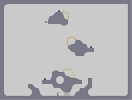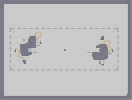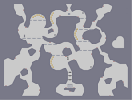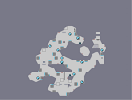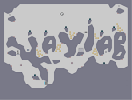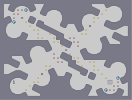When adventure becomes non-linear... Money in the gutter The Perfunctory Philosophy ~ 00-0 I lit a match, then let it catch... Ode to a high-five addict Rate Exchange Question Mark

WHOOOAAAA [=

u make epic N art!

^^

WICKED N-ART!

:D WOW :D

5/5 all his maps r 5/5

Well?

Did the season start? :)

all

N arts look better in thumbnails

LOL [nmaps.net]

:D

you have done better

Let's put it that way

It's okay,

But I wouldn't say great.
And gantrka is just jealous. He obviously has no idea how hard decent n-arts are to produce...

flawless :D

You artists and race makers get all the attention

but a solid action?
It seems like appreciation is lagging

It looks like...

Sexually Harassed Panda to me.

sexual harrasment Panda!

heh
I can see why they don't like having sex - look at that flab!

amazing

and this one is not fat its cuddly 5

For a fatty.

5/5

Absolutely incredible!
i thought it looked like skypanda
>_>

:/
5/5
its snorlax<3

This reminds me of something else funny.

This is the "Greatest survival ever," even though Panda's are least likely to survive anything endangering. That's why we have the PFP [nmaps.net] wildlife organization.

As long as you don't

change the name to Pandara's box, I'm fine with it XP.

playableness = 0

coolness = 10

avg = 5/5

Pandara's box!

Why the cheesy title?

I would've prefered something boring like "Panda" to something done to death like "Pandamonium"

Hahaha

gameplay= 0 rating
tiles= 0 rating

should i rate it as a 0?

ahhhh i would but i already gave it a 5. sorry

1 minus 1

5aved

hehe <3 Stepfather

I think he might have sniped but I dont care :D

stepfather

It's art. I don't know why you'd load it into your N when you can obviously see that it's an impossible map that is a panda made out of rockets. You amuse me.

what the hell

this is pointless and it looks crap when you load it up and it takes ages to load.

lovely

I try my best

who is guiseppi again?

vaguely remember him

Can someone tell Guiseppi

that I miss him? That would be great.

I hate to be a Skyray

but I honestly don't think this is worth all the fives it is getting.

F'ing awesome xD

He looks so fat

I dislike the stems coming off the bamboo and also the eyes. In general it seems a little shabby tbh

5